# ISEE Upper Level Quantitative : How to find the surface area of a prism

## Example Questions

### Example Question #1 : Prismsis a positive number. Which is the greater quantity?

(A) The surface area of a rectangular prism with length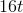, width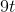, and height(B) The surface area of a rectangular prism with length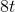, width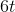, and height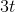.

It is impossible to determine which is greater from the information given

(A) is greater

(A) and (B) are equal

(B) is greater

(A) is greater

Explanation:

The surface area of a rectangular prism can be determined using the formula: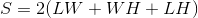Using substitutions, the surface areas of the prisms can be found as follows:

The prism in (A):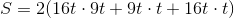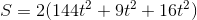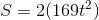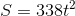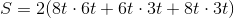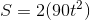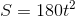Regardless of the value of,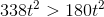- that is, the first prism has the greater surface area. (A) is greater.